# AP Physics 2 Practice Test 12

### Test Information10 questions18 minutes

1.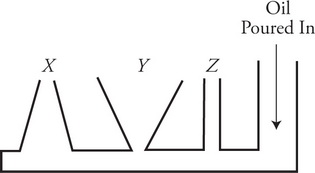Oil is very slowly poured into the container shown in the figure until the fluid enters the three open tubes X, Y, and Z. The oil does not overflow any of the tubes. Which of the following correctly ranks the height of the oil in each of the three tubes?

2. Two identical containers are filled with different gases. Container 1 is filled with hydrogen and container 2 is filled with nitrogen. Each container is set on a lab table and allowed to come to thermal equilibrium with the room. Which of the following correctly compares the properties of the two gases?

3.The graph shows the distribution of speeds for a gas sample at temperature T. The gas is heated to a higher temperature. Which of the following depicts a possible distribution after the gas has been heated? (Horizontal line N and vertical line S are shown for reference.)

4.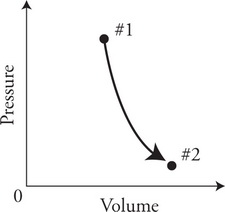The graph shows the pressure and volume of a gas being taken from state #1 to state #2, in a very quick process, where there is not enough time for heat to either leave or enter the gas. Which of the following correctly indicates the sign of the work done on the gas, and the change in temperature of the gas?

Work Done     ΔTemperature

5.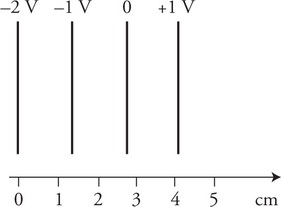In a region of space, there is an electric potential field with isolines as shown in the figure. Which of the following is the most accurate description of the electric field in the region?

6.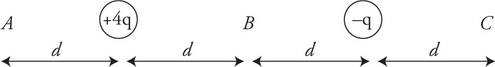Which of the following correctly represents the relationship between the electric field strengths at locations A, B, and C?

7.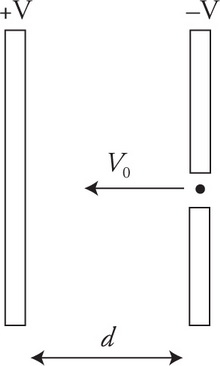An electron of charge -e and mass m is launched with a velocity of v0 through a small hole in the right plate of a parallel plate capacitor toward the opposite plate a distance d away. The electric potential of both plates are equal in magnitude, but opposite in sign ±V as shown in the figure. What is the kinetic energy of the electron as it reaches the left plate?

8.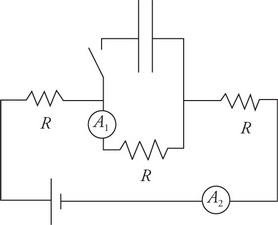The circuit shown in the figure consists of three identical resistors, two ammeters, a battery, a capacitor, and a switch. The capacitor is initially uncharged and the switch is open.

What happens to the readings of the ammeters immediately after the switch is closed?

A1     A2

9.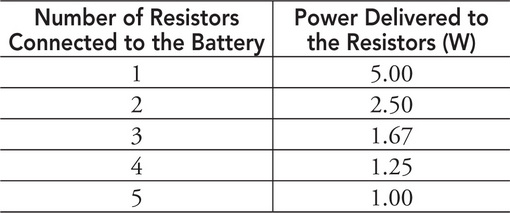A student connects a single resistor to a 10-V battery and takes measurements to find the power delivered by the battery to the resistor. The student then adds another resistor to the circuit and measures the power delivered to both resistors. This process is repeated, adding one resistor at a time, until the battery is connected to five resistors. The data from the experiment is given. Which of the following can be concluded from the data?

10. An electronics manufacturer needs a 1.2-&OMEGA; resistor for a phone it is designing. The company has calculated that it is less expensive to build the 1.2-&OMEGA; resistor from cheaper 1-&OMEGA; and 2-&OMEGA; resistors than to purchase the 1.2-&OMEGA; resistor from a supplier. Which of the following resistor arrangements is equivalent to 1.2 &OMEGA; and is the most effective method to construct the 1.2-&OMEGA; resistor?